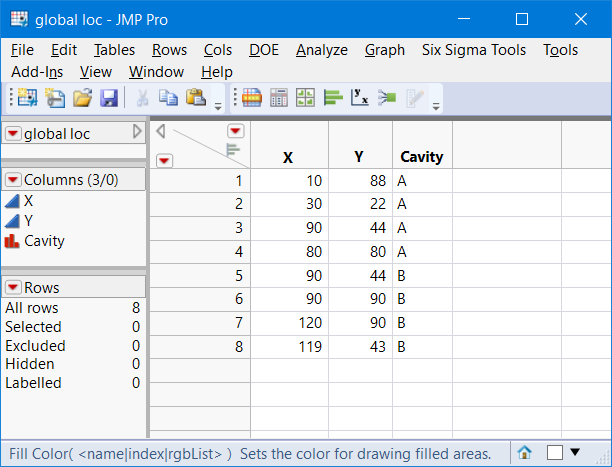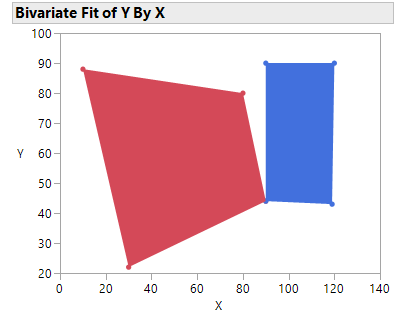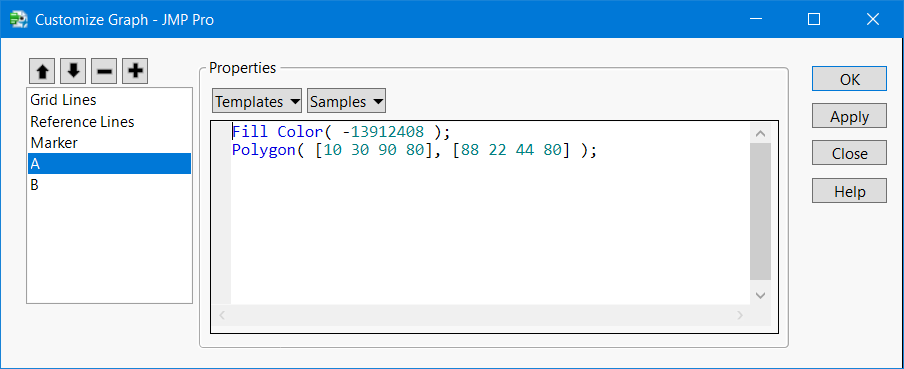Choose Language Hide Translation Bar
Highlighted

## JSL Graph Box Marker Select

I am trying to make the markers that have been placed in a Graph Box (using JSL) selectable. Currently, the graphic shows the markers, but I can't seem to select them - or the rows don't highlight in the data table.

Thoughts?

Thanks

1 ACCEPTED SOLUTION

Accepted Solutions

## Re: JSL Graph Box Marker Select

The attached table and script does is a concept script. It does not handle the offset and local vs global X and Y locations.

It creates the table``````Names Default To Here( 1 );

//create table
dt = New Table( "global loc",
New Column( "X",
Numeric,
"Continuous",
Format( "Best", 12 ),
Set Values( [10, 30, 90, 80, 90, 90, 120, 119] )
),
New Column( "Y",
Numeric,
"Continuous",
Format( "Best", 12 ),
Set Values( [88, 22, 44, 80, 44, 90, 90, 43] ),
Set Display Width( 51 )
),
New Column( "Cavity",
Character,
"Nominal",
Set Values( {"A", "A", "A", "A", "B", "B", "B", "B"} )
)
);

dt << Color by Column(:Cavity);

biv = dt << Bivariate( Y( :Y ), X( :X ) );

fb = report(biv)[FrameBox(1)];

cavList = Associative Array(dt:Cavity) << get keys;

for(i=1, i<=nitems(cavList), i++,
idx = dt << get rows where(:Cavity == cavList[i]);
xmat = dt:X[idx];
ymat = dt:Y[idx];
clr = color of(Row State (idx));
Eval(eval Expr(fb << Add Graphics Script( Description(Expr(cavList[i])),
Fill Color(Expr(clr));
Polygon( Expr(xmat`), Expr(ymat`))
)));
);
``````

Then adds a script for each polygon, rectanngle??Right click on the framebox and select customizeThere are multiple platforms to handle this.  Your problem is one similar to semiconductor wafer maps and alignment (mis-alignment) of layers.

Hope that helps. Now to select a shape you can use @markbailey suggestion also adding a row llegend can help with connectivity to the data table.

``fb << Row Legend( "Cavity", Color(1), Marker(0) );``

There is much more you can do. It depends upon the GUI you want.

5 REPLIES 5
Highlighted

## Re: JSL Graph Box Marker Select

You have to trap mouse actions on your own if you build a plot using a Graph Box. Alternatively, you could use a platform with a plot that is close to what you want, such as Bivariate or Graph Builder, and the dynamic linking is free.

Learn it once, use it forever!
Highlighted

## Re: JSL Graph Box Marker Select

A graph generated in a Graph Box() does not inherently have a connection to a data table and/or the selection of rows in the data table. Can you provide more details on the script, and even possibly attach the script to the discussion so the community can help with the resolution?
Jim
Highlighted

## Re: JSL Graph Box Marker Select

It would not be easy to supply the code.  But, maybe there is a platform I could use that would allow me two do two things: 1) plot many points based on their x-axis/y-axis values and 2) draw lines between specific sets of those same points.

Think about injection molding.  I have a multi-cavity mold, laid out in an x by y grid of cavities, where I am measuring the shape of each part for each cavity.  I am trying to show this information graphically where there are x * y parts and all measurements are relative to a 0,0 coordinate of the lower left corner of the mold.

Hope this helps to explain.

## Re: JSL Graph Box Marker Select

The attached table and script does is a concept script. It does not handle the offset and local vs global X and Y locations.

It creates the table``````Names Default To Here( 1 );

//create table
dt = New Table( "global loc",
New Column( "X",
Numeric,
"Continuous",
Format( "Best", 12 ),
Set Values( [10, 30, 90, 80, 90, 90, 120, 119] )
),
New Column( "Y",
Numeric,
"Continuous",
Format( "Best", 12 ),
Set Values( [88, 22, 44, 80, 44, 90, 90, 43] ),
Set Display Width( 51 )
),
New Column( "Cavity",
Character,
"Nominal",
Set Values( {"A", "A", "A", "A", "B", "B", "B", "B"} )
)
);

dt << Color by Column(:Cavity);

biv = dt << Bivariate( Y( :Y ), X( :X ) );

fb = report(biv)[FrameBox(1)];

cavList = Associative Array(dt:Cavity) << get keys;

for(i=1, i<=nitems(cavList), i++,
idx = dt << get rows where(:Cavity == cavList[i]);
xmat = dt:X[idx];
ymat = dt:Y[idx];
clr = color of(Row State (idx));
Eval(eval Expr(fb << Add Graphics Script( Description(Expr(cavList[i])),
Fill Color(Expr(clr));
Polygon( Expr(xmat`), Expr(ymat`))
)));
);
``````

Then adds a script for each polygon, rectanngle??Right click on the framebox and select customizeThere are multiple platforms to handle this.  Your problem is one similar to semiconductor wafer maps and alignment (mis-alignment) of layers.

Hope that helps. Now to select a shape you can use @markbailey suggestion also adding a row llegend can help with connectivity to the data table.

``fb << Row Legend( "Cavity", Color(1), Marker(0) );``

There is much more you can do. It depends upon the GUI you want.

Highlighted

## Re: JSL Graph Box Marker Select

@gzmorgan0 Thanks! This works and yes, it is siilar to semiconductor wafer maps.
Article Labels